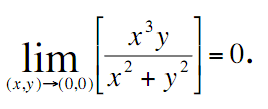# Multivariable Calculus - a question of limits

PhysicalProof

## Homework Statement

Prove the following, using the meaning of a limit:## Homework Equations

epsilon > 0, delta > 0

0 < sqrt(x^2 + y^2) < delta
| f(x) - 0 | < epsilon (1)

## The Attempt at a Solution

So, I know that I have to elaborate on the inequality in (1), further. However, I'm not sure of how to go about this. If I start transposing it seems to get much too messy and I end up with a proposed delta which seems far too complex. Is there some simplification that I'm overlooking here?

Any help is really appreciated, ta.

ninty
Could you not use polar coordinates instead?
Then the equation would be much simpler and the limit is just as r goes to 0

PhysicalProof
I did consider that but I'm pretty sure the limit definition must be used here (as a requirement).

Does anyone have any ideas of how to simplify this beast?

EDIT: Really need this one clarified guys. Does anyone have any hints at all? Even a vague idea.

Staff Emeritus